Checking if perfect cube

Chapter 6 Class 8 Cubes and Cube Roots
Concept wise

Let’s do this by examples

#### Is 216 a perfect cube?

Let’s do prime factorization of 216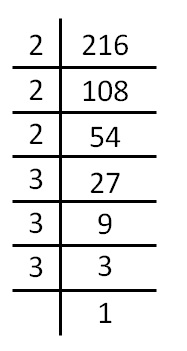Thus,

216  = 2 × 2 × 2 × 3 × 3 × 3

Now,

We make groups of 3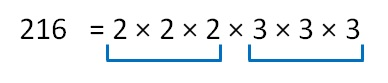Since 2 & 3 occur in triplets.

∴ 216 is a perfect cube.

Cube root of 216 = 2 × 3

= 6

### Is 729 a perfect cube?

Doing Prime factorization of 729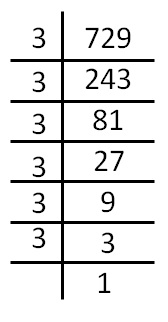We see that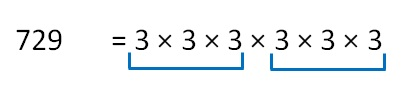Since 3 occurs in triplets.

∴ 729 is a perfect cube.

Cube root of 729 = 3 × 3

### Is 3375 a perfect cube?

Doing Prime factorization of 3375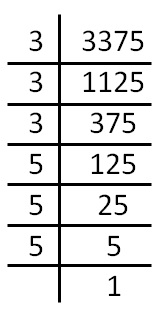We see that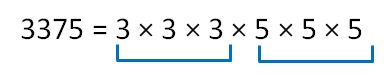Since 3 & 5 occurs in triplets.

∴ 3375 is a perfect cube.

Cube root of 3375 = 3 × 5

= 15

### Is 1125 a perfect cube?

Doing Prime factorization of 1125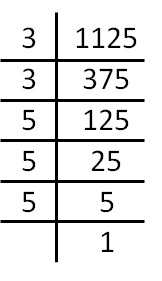We see that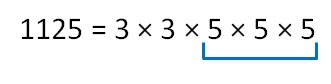Since 3 does not occur in triplets

∴ 1125 is not a perfect cube.

### Is 6859 a perfect cube?

Doing Prime factorization of 6859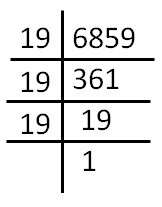We see that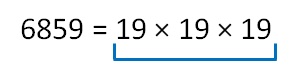Since 19 occurs in triplets.

∴ 6859 is a perfect cube.

Cube root of 6859 = 19

### Is 10648 a perfect cube?

Doing Prime factorization of 10648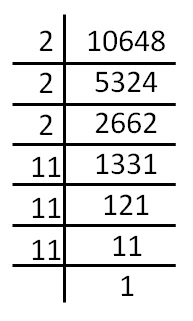We see that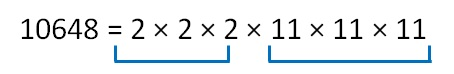Since 2 & 11 occurs in triplets.

∴ 10648 is a perfect cube.

Cube root of 10648 = 2 × 11

= 22

### Is 5324 a perfect cube?

Doing Prime factorization of 5324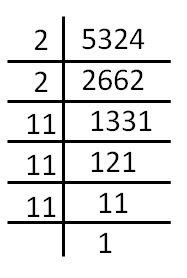We see that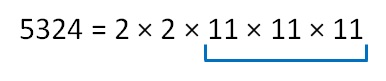Since 2 does not occur in triplets.

∴ 5324 is not a perfect cube.

### Is 968 a perfect cube?

Doing Prime factorization of 968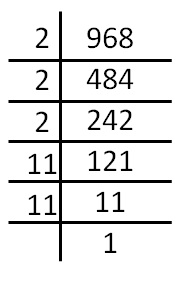We see that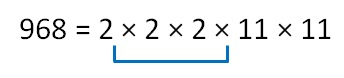Since 11 does not occur in triplets.

∴ 968 is not a perfect cube

### Is 400 a perfect cube?

Doing Prime factorization of 400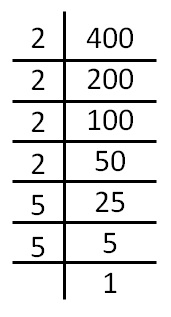Since 2 & 5 do not occur in triplets.

∴ 400 is not a perfect cube.

Learn in your speed, with individual attention - Teachoo Maths 1-on-1 Class